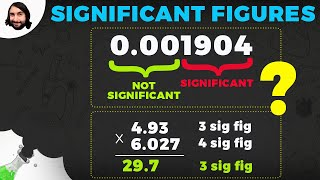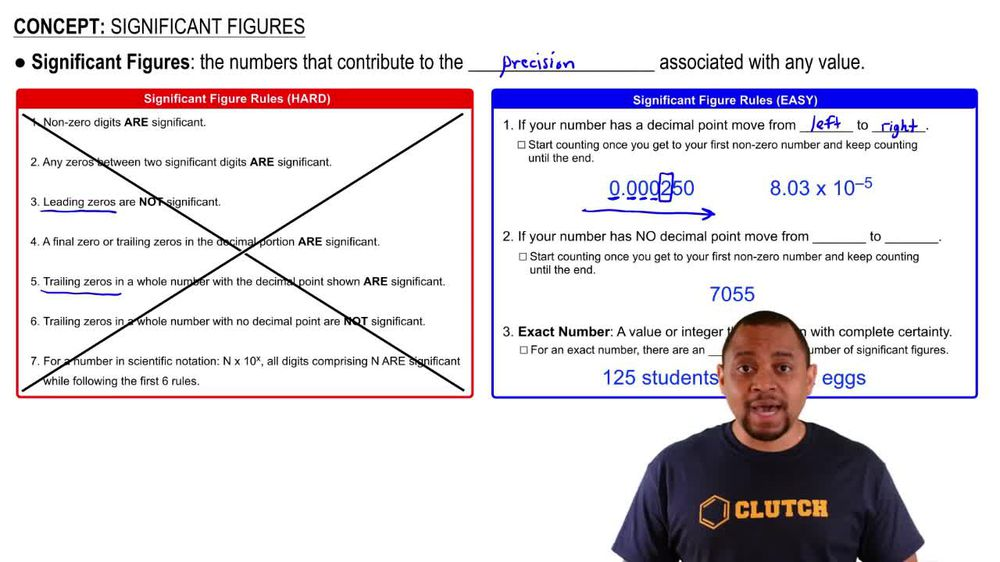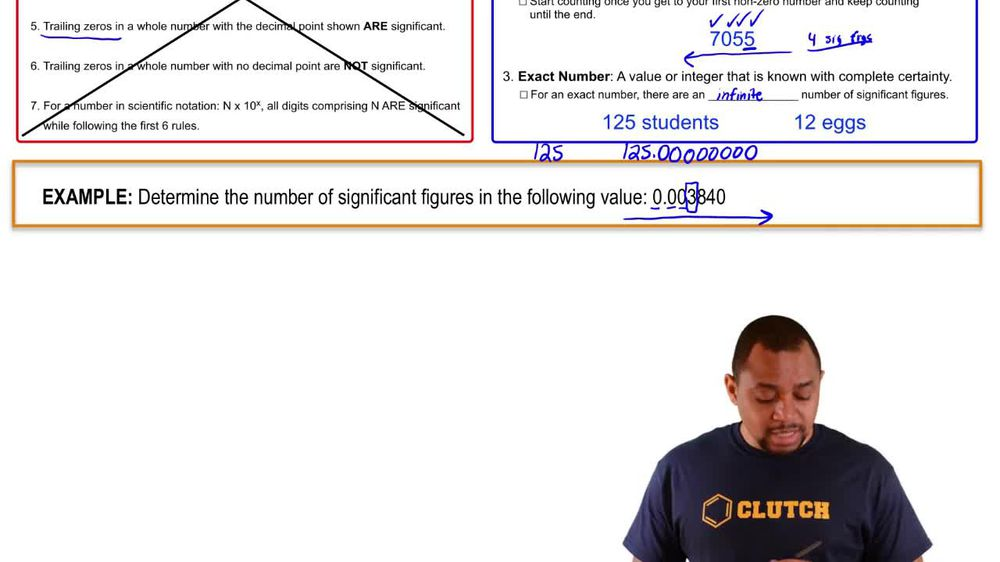Start typing, then use the up and down arrows to select an option from the list.
1. 1. Intro to General Chemistry2. Significant Figures
Problem

# (a) The diameter of Earth at the equator is 12756.27 km. Round this number to three significant figures and express it in standard exponential notation.

Relevant Solution1m
Play a video:
Hello everyone today. We are being given the following number here and being asked to write it and standard exponential notation that is also rounded off To two significant figures. The first thing we want to do when we have this number here is you want to rewrite this number with a different placement of the decimal point. We want to start by writing out the first Whole number which is two followed by a decimal point. And since it is Two significant figures it would just be 2.0. Whenever zeros followed by a decimal point And a whole number that is non-0, it is considered significant. So we see how many decimal places we move from the original decimal point in red. one, We have moved seven decimal places to the left. Therefore this would be 2.0 times 10 to the 7th power. And with that we have rewritten this number and standard exponential notation as well as in two significant figures. I hope this helped. And until next time.